# 视频学习

• 常用分类损失：交叉熵损失、hinge loss

• 常用回归损失：均方误差、平均绝对值误差（L1损失）

CNN解决方法：局部关联，参数共享

32×32×3 image → stretch to 3072×1

CNN典型结构

AlexNet（8 layers）：2012年ImageNet图像分类错误率比上年下降10%。

AlexNet成功原因：

• ImageNet大数据训练

• ReLU非线性激活函数

Sigmoid 小于-5大于5时梯度变为0，梯度消失无法更新网络。ReLU在一定程度上解决了梯度消失问题。

ReLU表达式简单，计算速度快。

ReLU收敛速度快，梯度比Sigmoid大。

• Dropout、Data augmentation防止过拟合

Dropout训练时随机关闭部分神经元，测试时整合所有神经元。

数据增强：随机裁剪、翻转、改变RGB通道强度等。

• 双GPU实现

ZFNet：2013年ImageNet分类冠军。错误率16.4% → 14.8%。

VGG（16-19）：2014年第二。AlexNet上加深深度。未有批归一化，先训练前11层固定参数，再训练后面的层。

Inception V2：增加1×1卷积核进行降维。

Inception V3：进一步对V2的参数数量进行降低。使用两个3×3卷积核替代5×5卷积核，降低了参数量。卷积核增多，非线性激活函数增加，表征能力更强，训练更快。

ResNet（152）：2015年三项竞赛冠军。

• 隐藏层的层数过多
• 采用了不合适的激活函数(更容易产生梯度消失，但是也有可能产生梯度爆炸)

• 隐藏层的层数过多
• 权重的初始化值过大

ResNet实现

CNN可以拟合任何一个函数。

ResNet由5个stage组成，每个stage有若干个block，每个block有若干个convs。可以实现可扩展性的代码。

50+/50-的ResNet，组成结构有什么不同：

50层以下的每个block由两个layer组成，没有BottleNeck。不降维的话，网络深度增加，参数量过大，高维时channel已经有256维。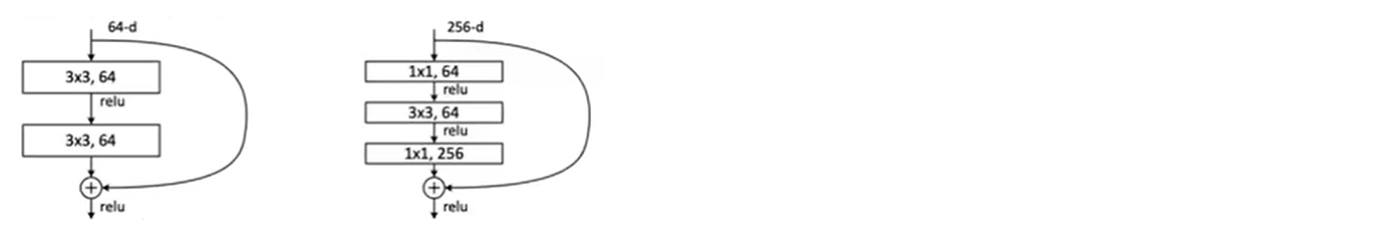↑ 注意细节，第一层直接调用relu，第二层先融合再relu。

ResNetXt 分组卷积

ResNetXt-Attention 注意力机制

ResNetXt WSL 弱监督训练

# 代码练习

• 加载数据

• root 为数据集下载到本地后的根目录，包括 training.pt 和 test.pt 文件
• train，如果设置为True，从training.pt创建数据集，否则从test.pt创建。
• transform, 一种函数或变换，输入PIL图片，返回变换之后的数据。
• target_transform 一种函数或变换，输入目标，进行变换。

transforms.Compose()

Compose里面的参数实际上就是个列表，而这个列表里面的元素就是想要执行的transform操作。

train_loader = torch.utils.data.DataLoader(
transform=transforms.Compose(
[transforms.ToTensor(),
transforms.Normalize((0.1307,), (0.3081,))])),
batch_size=64, shuffle=True)

datasets.MNIST('./data', train=False, transform=transforms.Compose([
transforms.ToTensor(),
transforms.Normalize((0.1307,), (0.3081,))])),
batch_size=1000, shuffle=True)

• 图片展示

plt.imshow(np.transpose(npimg, (1, 2, 0)))

因为在plt.imshow在实现的时候输入的是（imagesize,imagesize,channels），而def imshow(img)中，参数img的格式为（channels,imagesize,imagesize），这两者的格式不一致，我们需要调用一次np.transpose函数，即np.transpose(npimg,(1,2,0))，将npimg的数据格式由（channels,imagesize,imagesize）转化为（imagesize,imagesize,channels），进行格式的转换后方可进行显示。

def imshow(img):
plt.figure(figsize=(8,8))
img = img / 2 + 0.5     # 转换到 [0,1] 之间
npimg = img.numpy()
plt.imshow(np.transpose(npimg, (1, 2, 0)))
plt.show()

• Net计算

class Net(nn.Module):
def __init__(self):
super(Net, self).__init__()
self.conv1 = nn.Conv2d(3, 6, 5)     #32*32*3  28*28*6
self.pool = nn.MaxPool2d(2, 2)      #14*14*6
self.conv2 = nn.Conv2d(6, 16, 5)    #10*10*16
#self.pool = nn.MaxPool2d(2, 2)     #5*5*16
self.fc1 = nn.Linear(16 * 5 * 5, 120)
self.fc2 = nn.Linear(120, 84)
self.fc3 = nn.Linear(84, 10)


# 论文学习

Inception V1

• 为什么要多个不同大小的卷积核并行？

• 1×1卷积起到降维操作。

• 辅助分类器

层数加深后会难以训练出现梯度消失问题，后面的变化很难反向传播。辅助分类器可能起到一个类似逐层预训练的作用。

辅助分类器是将中间某一层的输出用作分类，并按一个较小的权重（0.3）加到最终分类结果中，这样相当于做了模型融合，同时给网络增加了反向传播的梯度信号，也提供了额外的正则化。而在实际测试的时候，这两个额外的softmax会被去掉。

Inception V3

• 卷积分解

使用两个3×3卷积核替代5×5卷积核，感受野不变，降低了参数量。卷积核增多，非线性激活函数增加，表征能力更强，训练更快。

任意nxn的卷积都可以通过1xn卷积后接nx1卷积来替代。在网络的前期使用这种分解效果并不好，在中度大小的特征图上使用效果才会更好（特征图大小建议在12到20之间）。

• 高效的降维

池化并行。先池化再Inception卷积，或者先Inception卷积再作池化。但是先作池化会导致特征缺失，先作卷积是正常的缩小，但计算量很大。为了同时保持特征表示且降低计算量，将网络结构改为使用两个并行化的模块来降低计算量（卷积、池化并行执行，再进行通道合并）。

Inception V4

• 加入了残差学习

• 为什么加了残差后可以解决梯度消失问题

拟合H(x)，由于网络退化问题难以训练出来。转为训练F(x)，F(x)=H(x)-x，F(x)称为残差，然后H(x)=F(x)+x。

学习H(x)和F(x)的差，更容易学习，就像画一个人在有人物轮廓的情况下加细节，会容易些。

MobileNets: Efficient Convolutional Neural Networks for Mobile Vision Applications

• 常规卷积操作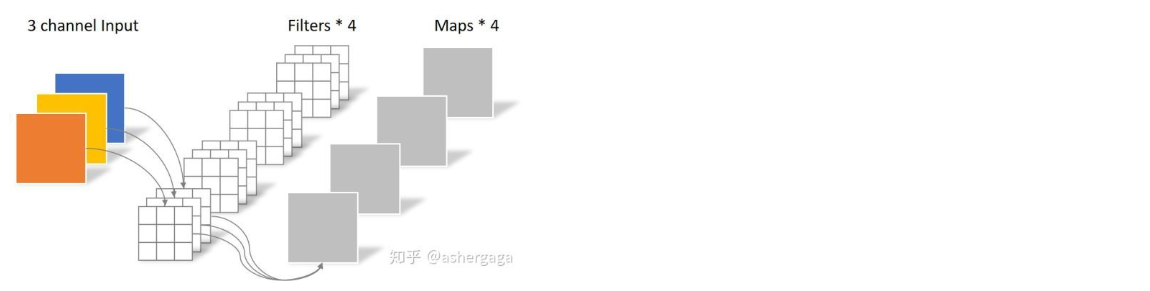• 深度可分离卷积

• 逐通道卷积（Depthwise Convolution）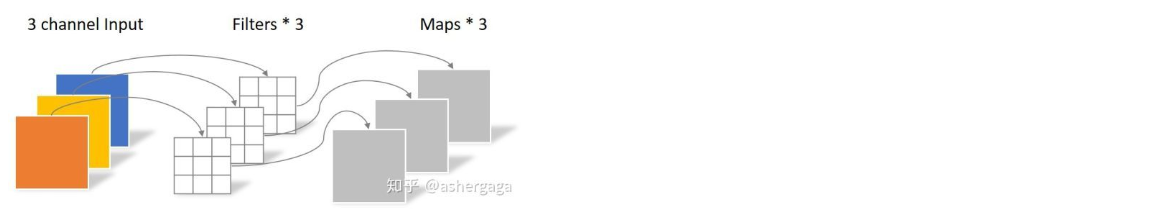DW是做了分组卷积（group convolution），做了一个特征的分组，对feature map进行分组可以显著提高准确率。

• 逐点卷积（Pointwise Convolution）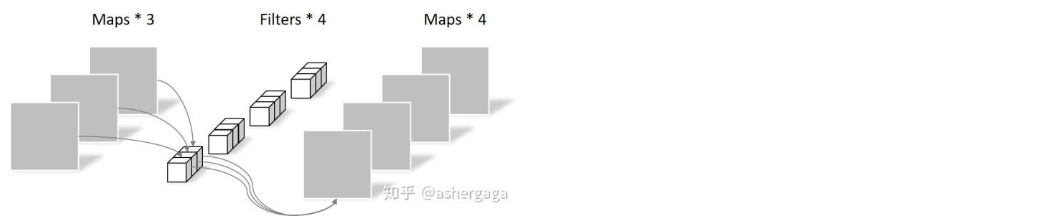用1×1的卷积进行升维操作。

同样的输入，同样得到4张feature map，参数量显著减少。

HybridSN: Exploring 3-D–2-D CNN Feature Hierarchy for Hyperspectral Image Classification

• 高光谱图像：由二维空间信息和一维光谱信息组成。

• 二维卷积神经网络的问题：不能充分提取高光谱图像的光谱信息，只能提取三个通道。
三维卷积神经网络的问题：可以提取到光谱信息，但增加了计算复杂度。

• 高光谱图像光谱通道数过多，直接进行计算的话运算缓慢。先进行降维，PCA降维到30/15个通道即可保留到99%以上的信息。

• 结构：三层三维卷积神经网络＋一层二维卷积神经网络+三层全连接。三维提取空间信息和光谱信息，二维进一步提取空间信息。

• 二维卷积和三维卷积的区别：二维卷积和特征图大小没有关系，输入100×100或200×200都可以，但要考虑输入的通道数。三维卷积可以无视输入的通道数。

• 思考：用什么方法可以进一步提高网络性能？
考虑把二维卷积替换成mobilenets，至少可以降低计算复杂度。

# 纹理缺陷检测尝试

import numpy as np
import matplotlib.pyplot as plt
import os
import torch
import torch.nn as nn
import torchvision
from torchvision import models,transforms,datasets
import time
import json
import cv2
import csv

# 判断是否存在GPU设备
device = torch.device("cuda:0" if torch.cuda.is_available() else "cpu")
print('Using gpu: %s ' % torch.cuda.is_available())

image_height = 512
image_width = 512


file_dir = '/content/drive/My Drive/AIyanxishe80'

with open(file_dir + '/train.csv', 'r') as f:
#print(result)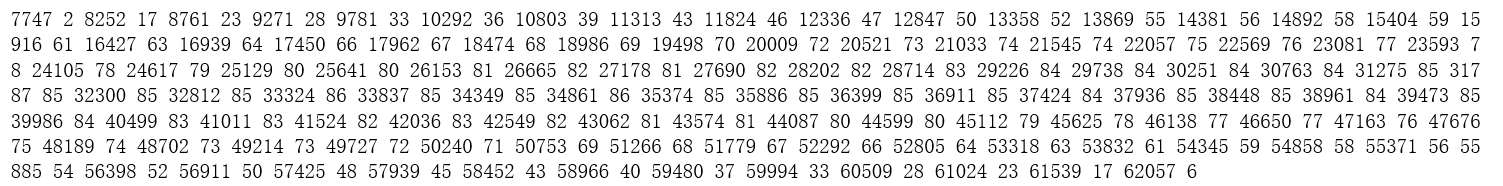def rle_decode(mask_rle: str = '', shape: tuple = (1400, 2100)):
starts, lengths = [np.asarray(x, dtype=int) for x in (s[0:][::2], s[1:][::2])]
starts -= 1
ends = starts + lengths
img = np.zeros(shape * shape, dtype=np.uint8)
for lo, hi in zip(starts, ends):#进行恢复
img[lo:hi] = 255
return img.reshape(shape, order='F')

array = rle_decode(result,(image_height,image_width))
cv2_imshow(array)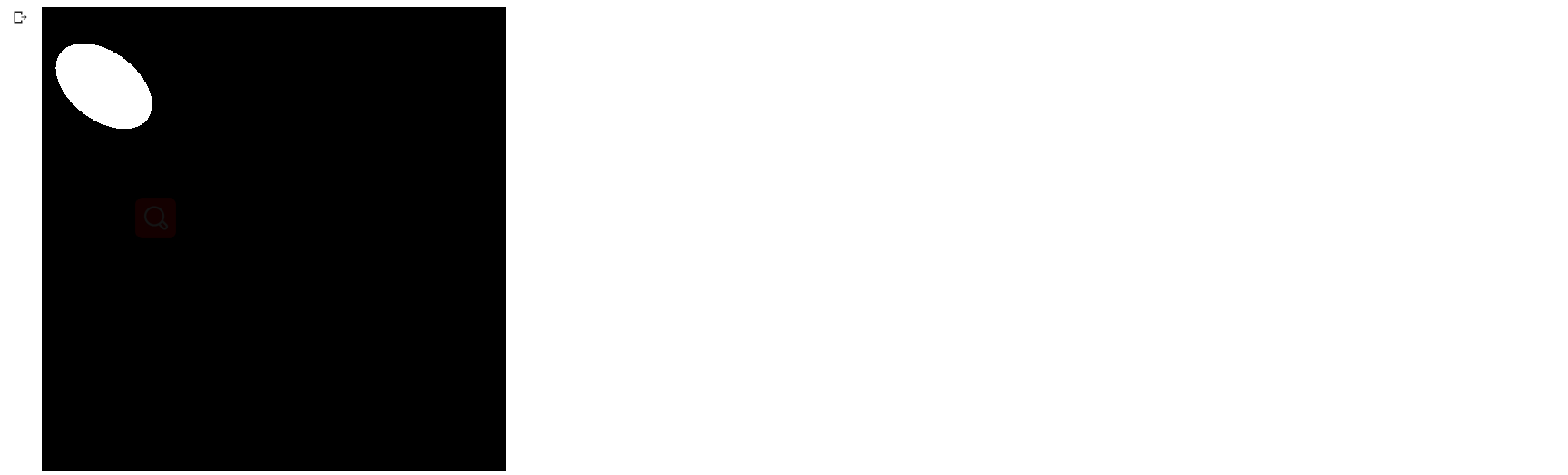def draw_mask_edge_on_image_cv2(image, mask):
image = cv2.cvtColor(image,cv2.COLOR_RGB2GRAY)
contours, _ = cv2.findContours(mask, cv2.RETR_TREE, cv2.CHAIN_APPROX_SIMPLE)
x, y, w, h = cv2.boundingRect(contours)
image = cv2.cvtColor(image, cv2.COLOR_GRAY2BGR)
cv2.rectangle(image, (x, y), (x+w, y+h), (255, 255, 255), 1)
#cv2.drawContours(image, contours, -1, (0, 0, 255), 1)
return image, x, y, w, h

array = rle_decode(result,(image_height,image_width))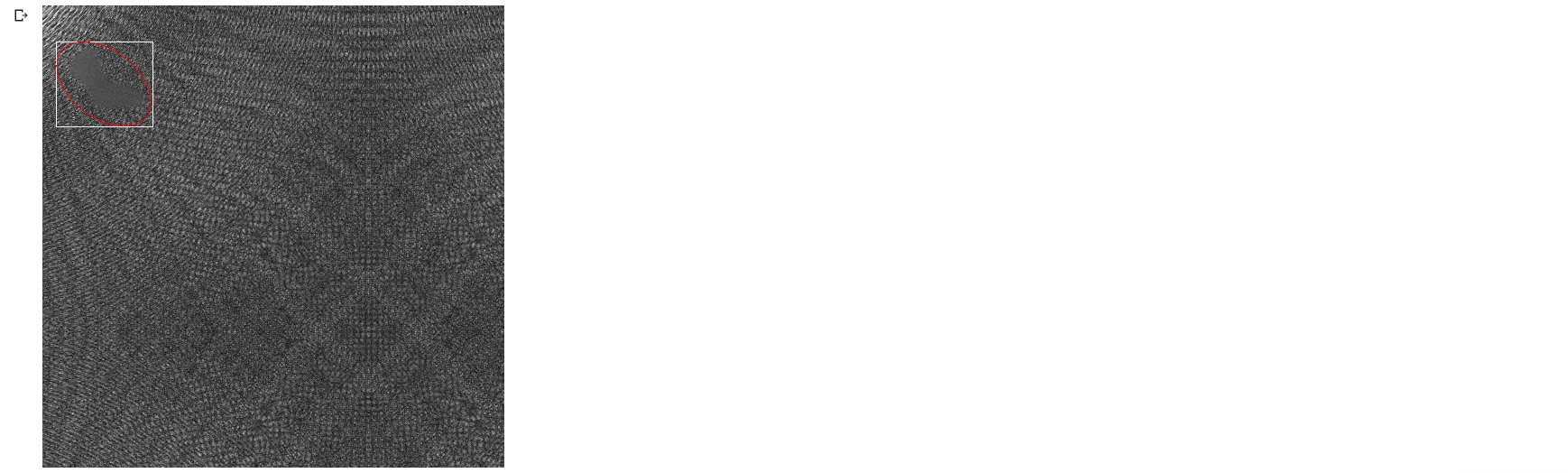array = rle_decode(result,(image_height,image_width))
image = cv2.imread(file_dir + '/Train/' + str(0) + '.png')
img_a = torch.from_numpy(image).unsqueeze(0)
y_a = torch.tensor([[x,y,w,h]])

for i in range(1886):
array = rle_decode(result[i+2],(image_height,image_width))
image = cv2.imread(file_dir + '/Train/' + str(i+1) + '.png')
img_b = torch.from_numpy(image).unsqueeze(0)
img_a = torch.cat((img_a,img_b), 0).type(torch.FloatTensor)
y_b = torch.tensor([[x,y,w,h]])
y_a = torch.cat((y_a,y_b), 0).type(torch.FloatTensor)
print("[ %.2f / 100 ]" % ((i+2)*100/1886))
display.clear_output(wait=True)

X = img_a
y = y_a

dataset = Data.TensorDataset(X, y)


posted @ 2020-08-01 20:03  薄暮  阅读(43)  评论(0编辑  收藏